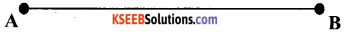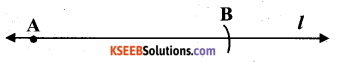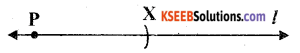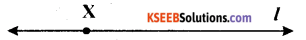# KSEEB Solutions for Class 6 Maths Chapter 14 Practical Geometry Ex 14.2

Students can Download Chapter 14 Practical Geometry Ex 14.2 Questions and Answers, Notes Pdf, KSEEB Solutions for Class 6 Maths helps you to revise the complete Karnataka State Board Syllabus and score more marks in your examinations.

## Karnataka State Syllabus Class 6 Maths Chapter 14 Practical Geometry Ex 14.2

Question 1.
Draw a line segment of length 7.3 cm using a ruler.
Solution:
A line segment of length 7.3 cm can be drawn using a ruler as follows.
(1) Mark a point A on the sheet.
(2) Put 0 mark of ruler at point A.
(3) Mark a point B on the sheet at 7.3 cm on ruler.
(4) Join A and B.
$$\overline{\mathrm{AB}}$$ is the required line segment.Question 2.
Construct a line segment of length 5.6 cm using ruler and compasses.
Solution:
A line segment of length 5.6 cm can be drawn using a ruler and compasses as follows.
(1) Draw a line 1 and mark a point A on this line.(2) Place the compasses on the zero mark of the ruler. Open it to place the pencil up to the 5.6 cm mark.
(3) Place the pointer of compasses on point A and draw an arc to cut 1 at B. AB is the line segment of 5.6 cm length.Question 3.
Construct $$\overline{\mathbf{A B}}$$ of length 7.8 cm. From this, cut off $$\overline{\mathbf{A C}}$$ of length 4.7 cm. Measure BC.
Solution:
(1) Draw a line land mark a point A on it.
(2) By adjusting the compasses up to 7.8 cm, draw an arc to cut 1 on B, while putting the
pointer of compasses on point A.
$$\overline{\mathbf{A B}}$$ is the line segment of 7.8 cm.
(3) By adjusting the compasses up to 4.7 cm. draw an arc to cut 1 on C, while putting the pointer of compasses on point A. $$\overline{\mathbf{A C}}$$ is the line segment of4.7 cm.
(4) Now, put the ruler along with this line such that 0 mark of the ruler will match with point C. On reading the position of point B, it comes to 3.1 cm, $$\overline{\mathbf{B C}}$$ is 3.1 cmQuestion 4.
Given $$\overline{\mathbf{A B}}$$ of length 3.9 cm, construct $$\overline{\mathbf{P Q}}$$ such that the length of $$\overline{\mathbf{P Q}}$$ is twice that of $$\overline{\mathbf{A B}}$$. Verify by measurement.(Hint: Construct $$\overline{\mathbf{P X}}$$ such that length of $$\overline{\mathbf{P X}}$$ = length of $$\overline{\mathbf{A B}}$$ ; then cut off $$\overline{\mathbf{X Q}}$$ such that $$\overline{\mathbf{X Q}}$$ also has the length of $$\overline{\mathbf{A B}}$$.)
Solution:
A line segment $$\overline{\mathbf{P Q}}$$ can be drawn such that the length $$\overline{\mathbf{P Q}}$$ of is twice that of as follows.
(1) Draw a line 1 and mark a point P on it and let AB be the given line segment of 3.9 cm.(2) By adjusting the compasses up to the length of AB. draw an arc to cut the line at X, while taking the pointer of compasses at point P.(3) Again put the pointer on point X and draw an arc to cut line 1 again at Q.$$\overline{\mathbf{P Q}}$$ is the required line segment. By ruler, the length of $$\overline{\mathbf{P Q}}$$ can be measured which comes to 7.8 cm.Question 5.
Given $$\overline{\mathbf{A B}}$$ of length 7.3 cm and $$\overline{\mathbf{C D}}$$ of length 3.4 cm, construct a line segment $$\overline{\mathbf{X Y}}$$ such that the length of $$\overline{\mathbf{X Y}}$$ is equal to the difference between the lengths of
$$\overline{\mathbf{A B}}$$ and $$\overline{\mathbf{C D}}$$. Verify by measurement.
Solution:
(1) Given that, $$\overline{\mathbf{A B}}$$ = 7.3 cm and $$\overline{\mathbf{C D}}$$ = 3.4 cm(2) Adjust the compasses up to the length of CD and put the pointer of the compasses at A. Draw an arc to cut AB at P.(3) Adjust the compasses up to the length of PB. Now draw a line l and mark a point X on it.(4) Now, putting the pointer of compasses at point X, draw an arc to cut the line at Y.$$\overline{\mathbf{X Y}}$$ is the required line segment.

error: Content is protected !!